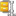Author Message m.hengstlerGroup: Forum Members Posts: 7, Visits: 1 Hi,I'm trying to program a pacman-like task in Inquisit.The first thing I would like to get to work is that Inquisit responds to key presses of the participants. So if a participants decides to go left (ie press left arrow) the pacman would turn left. If no response has been made by the participant, the pacman-figure should move in the way it was going. So, in short, a partipants controls the direction in which the figure is going, and the figure itself is moving by itself.I already managed to let the pacman move by itself, and I also managed to program that it responds to the key presses. However, on some occasions the pacman doesn't respond to the key press, presumably because it was made at exactly a loop-point. So basically it works, only that sometimes the pacman doesn't respond.So, my question would be: Is there a way that a pacman-figure is moving on its own, and a participant can intervene this movement by changing directions via key presses?Thanks in advance,MaikelPS: I've attached the script and figures. AttachmentsExperiment_pacman.zip (619 views, 27.00 KB) Daveposted 12 Years Ago ANSWERGroup: Administrators Posts: 12K, Visits: 95K However, on some occasions the pacman doesn't respond to the key press, presumably because it was made at exactly a loop-point.You probably won't be able to avoid this completely, however with the right timing, these occasions should be rare.So, my question would be: Is there a way that a pacman-figure is moving on its own, and a participant can intervene this movement by changing directions via key presses?You'll want to do something like this:/ windowsize = (800px, 600px)/ h = 50%/ v = 50%/ step = 2%/ direction = 205/ shape = circle/ size = (25px,25px)/ hposition = values.h/ vposition = values.v/ color = (white)/ erase = false/ shape = rectangle/ size = (100%,100%)/ color = (black)/ erase = false/ ontrialend = [if(trial.mytrial.response!=0)values.direction=trial.mytrial.response]/ ontrialend = [if(values.direction==200)values.v=values.v-values.step]/ ontrialend = [if(values.direction==208)values.v=values.v+values.step]/ ontrialend = [if(values.direction==205)values.h=values.h+values.step]/ ontrialend = [if(values.direction==203)values.h=values.h-values.step]/ ontrialend = [if(values.h<0)values.h=100%]/ ontrialend = [if(values.h>100%)values.h=0%]/ ontrialend = [if(values.v<0)values.v=100%]/ ontrialend = [if(values.v>100%)values.v=0%]/ stimulusframes = [1=mybackground, mydot]/ validresponse = (0,200,203,205,208)/ trialduration = 300/ branch = [trial.mytrial]/ trials = [1=mytrial]Regards,~Dave m.hengstlerGroup: Forum Members Posts: 7, Visits: 1 Thanks for your response. This programming is more straightforward indeed.Still sometimes it doesn't respond on the presses, so if anyone has an idea on that, please let me know.I'm now trying to program some 'detection-part', that is when two figures collide when moving (e.g. pacman catches a ghost or vice versa).

#### Merge Selected

Merge into selected topic...

Merge into merge target...

Merge into a specific topic ID...